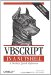# Section C.5. Operator Precedence

### C 5 Operator Precedence

If you include more than one operator in a single line of code, you need to know the order in which VBScript will evaluate them. Otherwise, the results may be completely different than you intend. The rules that define the order in which a language handles operators is known as the order of precedence. If the order of precedence results in operations being evaluated in an order other than the one you intendand therefore if the value that results from these operations is "wrong" from your point of viewyou can explicitly override the order of precedence through the use of parentheses. However, the order of precedence still applies to multiple operators within parentheses.

When a single line of code includes operators from more than one category, they are evaluated in the following order:

1. Arithmetic operators
2. Concatenation operators
3. Comparison operators
4. Logical operators

Within each category of operators except for the single concatenation operator, there is also an order of precedence. If multiple comparison operators appear in a single line of code, they are simply evaluated from left to right. The order of precedence of arithmetic operators is as follows:

1. Exponentiation (^)
2. Division and multiplication (/,*) (No order of precedence between the two)
3. Integer division ()
4. Modulo arithmetic (Mod)
5. Addition and subtraction (+,-) (No order of precedence between the two)

If the same arithmetic operator is used multiple times in a single line of code, the operators are evaluated from left to right.

The order of precedence of logical operators is:

1. Not
2. And
3. Or
4. Xor
5. Eqv
6. Imp

If the same arithmetic or logical operator is used multiple times in a single line of code, the operators are evaluated from left to right.VBScript in a Nutshell, 2nd Edition
ISBN: 0596004885
EAN: 2147483647
Year: 2003
Pages: 335